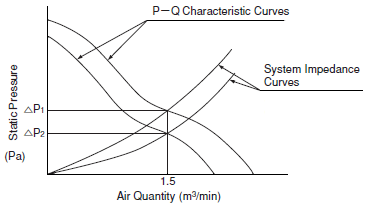DC fan motors

# 2. Selection of the Fan

The selection of the fan must be made as follows:

 a) Estimate the calorific value in the unit.b) Determine the temperature rise value in the unit. c) Calculate the needed air quantity. Q = 50 × Ha △T H   : Calorific value (kW) △T : Temperature rise value (deg.C) d) Estimate the system impedance in the unit. △P = KQn(Pa) △P : Reduced pressure (m3/min) K : Constant measured in each unit. Q : Air quantity N : Determined by the air flow in the unit. n = 1 for steady flow, n = 2 for turbulent flow. n = 2 may be generally used. e) Select the fan by the P-Q characteristics in the fan catalog. f) Mount it on the equipment to measure the temperature rise.

## Example: When setting the temperature rise value T = 10deg.C at calorific value 300WQ =

50 × 0.3
10

= 1.5 (m3/min)

Estimate the system impedance, select the fan, conduct the actual operation, measure the temperature rise and investigate the fan.